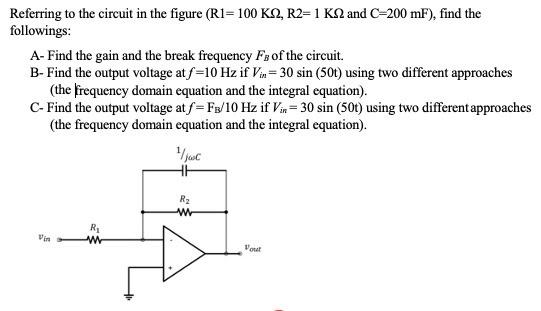# Question Hello, please make sure you understand this topic. make sure answers and formulas applied are correct. show the steps and answers. if answers are correct i will give thumbs up. Thank You! Referring to the circuit in the figure (R1=100 KS2, R2=1 K2 and C-200 mF), find the followings: A- Find the gain and the break frequency Fs of the circuit. B- Find the output voltage at f=10 Hz if Vin= 30 sin (50t) using two different approaches (the frequency domain equation and the integral equation). C- Find the output voltage at f=Fg/10 Hz if Vin= 30 sin (50t) using two different approaches (the frequency domain equation and the integral equation). oc HE RE w R w 5 VonatPLEWFB The Asker · Electrical Engineering
Hello, please make sure you understand this topic. make sure answers and formulas applied are correct. show the steps and answers. if answers are correct i will give thumbs up. Thank You!Transcribed Image Text: Referring to the circuit in the figure (R1=100 KS2, R2=1 K2 and C-200 mF), find the followings: A- Find the gain and the break frequency Fs of the circuit. B- Find the output voltage at f=10 Hz if Vin= 30 sin (50t) using two different approaches (the frequency domain equation and the integral equation). C- Find the output voltage at f=Fg/10 Hz if Vin= 30 sin (50t) using two different approaches (the frequency domain equation and the integral equation). oc HE RE w R w 5 Vonat
More
Transcribed Image Text: Referring to the circuit in the figure (R1=100 KS2, R2=1 K2 and C-200 mF), find the followings: A- Find the gain and the break frequency Fs of the circuit. B- Find the output voltage at f=10 Hz if Vin= 30 sin (50t) using two different approaches (the frequency domain equation and the integral equation). C- Find the output voltage at f=Fg/10 Hz if Vin= 30 sin (50t) using two different approaches (the frequency domain equation and the integral equation). oc HE RE w R w 5 Vonat